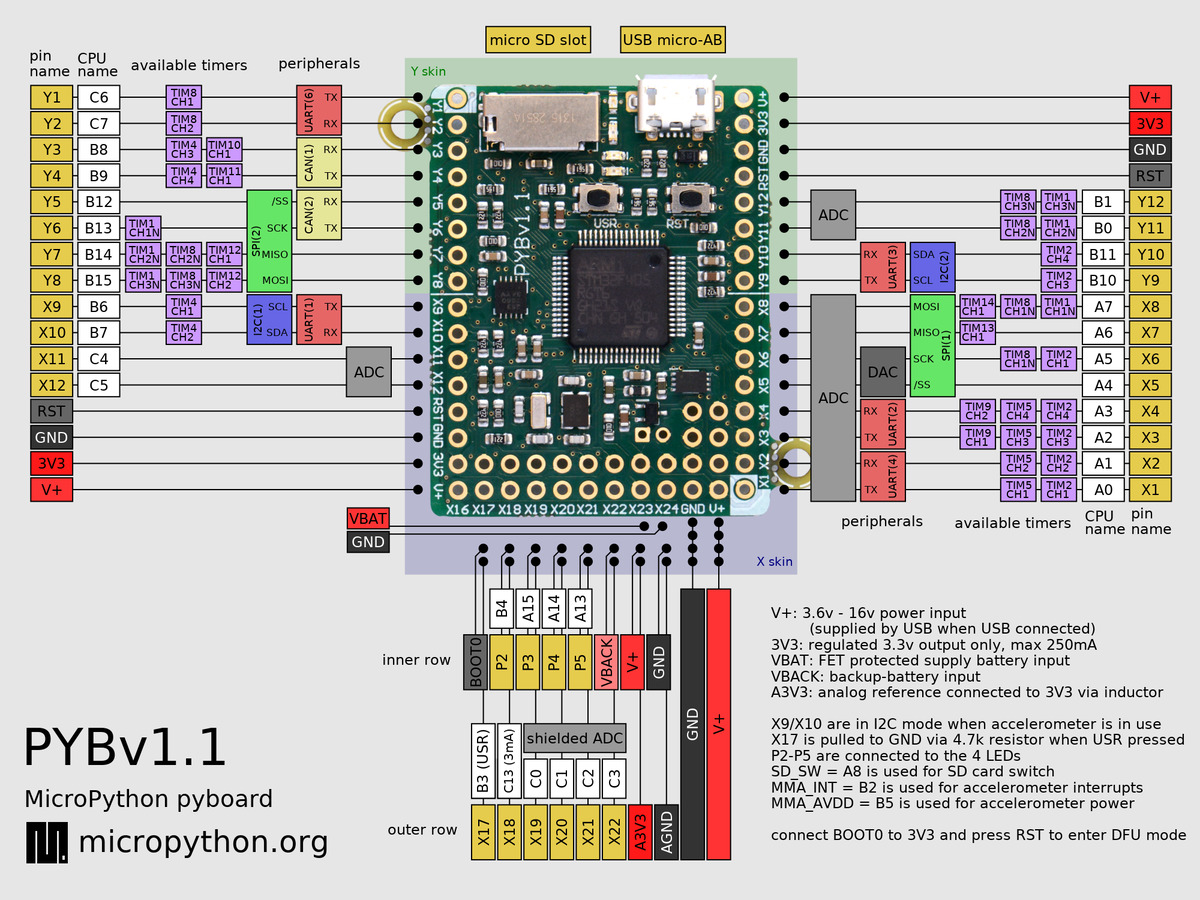# pyboard 快速参考 ¶## 一般板控制 ¶

```import pyb
pyb.repl_uart(pyb.UART(1, 9600)) # duplicate REPL on UART(1)
pyb.wfi() # pause CPU, waiting for interrupt
pyb.freq() # get CPU and bus frequencies
pyb.freq(60000000) # set CPU freq to 60MHz
pyb.stop() # stop CPU, waiting for external interrupt
```

## 延迟和计时 ¶

```import time
time.sleep(1)           # sleep for 1 second
time.sleep_ms(500)      # sleep for 500 milliseconds
time.sleep_us(10)       # sleep for 10 microseconds
start = time.ticks_ms() # get value of millisecond counter
delta = time.ticks_diff(time.ticks_ms(), start) # compute time difference
```

## 内部 LED ¶

pyb.LED .

```from pyb import LED
led = LED(1) # 1=red, 2=green, 3=yellow, 4=blue
led.toggle()
led.on()
led.off()
# LEDs 3 and 4 support PWM intensity (0-255)
LED(4).intensity()    # get intensity
LED(4).intensity(128) # set intensity to half
```

## 内部开关 ¶

```from pyb import Switch
sw = Switch()
sw.value() # returns True or False
sw.callback(lambda: pyb.LED(1).toggle())
```

## 引脚和 GPIO ¶

pyb.Pin .

```from pyb import Pin
p_out = Pin('X1', Pin.OUT_PP)
p_out.high()
p_out.low()
p_in = Pin('X2', Pin.IN, Pin.PULL_UP)
p_in.value() # get value, 0 or 1
```

## 伺服控制 ¶

```from pyb import Servo
s1 = Servo(1) # servo on position 1 (X1, VIN, GND)
s1.angle(45) # move to 45 degrees
s1.angle(-60, 1500) # move to -60 degrees in 1500ms
s1.speed(50) # for continuous rotation servos
```

## 外部中断 ¶

```from pyb import Pin, ExtInt
callback = lambda e: print("intr")
ext = ExtInt(Pin('Y1'), ExtInt.IRQ_RISING, Pin.PULL_NONE, callback)
```

## 计时器 ¶

```from pyb import Timer
tim = Timer(1, freq=1000)
tim.counter() # get counter value
tim.freq(0.5) # 0.5 Hz
tim.callback(lambda t: pyb.LED(1).toggle())
```

## RTC (实时时钟) ¶

```from pyb import RTC
rtc = RTC()
rtc.datetime((2017, 8, 23, 1, 12, 48, 0, 0)) # set a specific date and time
rtc.datetime() # get date and time
```

## PWM (脉冲宽度调制) ¶

pyb.Pin and pyb.Timer .

```from pyb import Pin, Timer
p = Pin('X1') # X1 has TIM2, CH1
tim = Timer(2, freq=1000)
ch = tim.channel(1, Timer.PWM, pin=p)
ch.pulse_width_percent(50)
```

```from pyb import Pin, ADC
```

## DAC (数字模拟转换) ¶

pyb.Pin and pyb.DAC .

```from pyb import Pin, DAC
dac = DAC(Pin('X5'))
dac.write(120) # output between 0 and 255
```

## UART (串行总线) ¶

pyb.UART .

```from pyb import UART
uart = UART(1, 9600)
uart.write('hello')
```

## SPI 总线 ¶

pyb.SPI .

```from pyb import SPI
spi = SPI(1, SPI.CONTROLLER, baudrate=200000, polarity=1, phase=0)
spi.send('hello')
spi.recv(5) # receive 5 bytes on the bus
spi.send_recv('hello') # send and receive 5 bytes
```

## I2C 总线 ¶

Hardware I2C is available on the X and Y halves of the pyboard via ``` I2C('X') ``` and ``` I2C('Y') ``` . Alternatively pass in the integer identifier of the peripheral, eg ``` I2C(1) ``` . Software I2C is also available by explicitly specifying the ``` scl ``` and ``` sda ``` pins instead of the bus name. For more details see machine.I2C .

```from machine import I2C
i2c = I2C('X', freq=400000)                 # create hardware I2c object
i2c = I2C(scl='X1', sda='X2', freq=100000)  # create software I2C object
i2c.scan()                          # returns list of peripheral addresses
i2c.writeto(0x42, 'hello')          # write 5 bytes to peripheral with address 0x42
i2c.readfrom_mem(0x42, 0x10, 2)     # read 2 bytes from peripheral 0x42, peripheral memory 0x10
i2c.writeto_mem(0x42, 0x10, 'xy')   # write 2 bytes to peripheral 0x42, peripheral memory 0x10
```

## I2S bus ¶

```from machine import I2S, Pin
i2s = I2S(2, sck=Pin('Y6'), ws=Pin('Y5'), sd=Pin('Y8'), mode=I2S.TX, bits=16, format=I2S.STEREO, rate=44100, ibuf=40000) # create I2S object
i2s.write(buf)             # write buffer of audio samples to I2S device
i2s = I2S(1, sck=Pin('X5'), ws=Pin('X6'), sd=Pin('Y4'), mode=I2S.RX, bits=16, format=I2S.MONO, rate=22050, ibuf=40000) # create I2S object
i2s.readinto(buf)          # fill buffer with audio samples from I2S device
```

The I2S class is currently available as a Technical Preview. During the preview period, feedback from users is encouraged. Based on this feedback, the I2S class API and implementation may be changed.

PYBv1.0/v1.1 has one I2S bus with id=2. PYBD-SFxW has two I2S buses with id=1 and id=2. I2S is shared with SPI.

## CAN (控制器区域网络) 总线 ¶

pyb.CAN .

```from pyb import CAN
can = CAN(1, CAN.LOOPBACK)
can.setfilter(0, CAN.LIST16, 0, (123, 124, 125, 126))
can.send('message!', 123)   # send a message with id 123
can.recv(0)                 # receive message on FIFO 0
```

## 内部加速度计 ¶

```from pyb import Accel
accel = Accel()
print(accel.x(), accel.y(), accel.z(), accel.tilt())
```